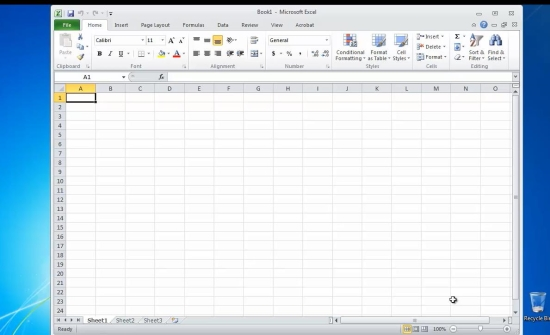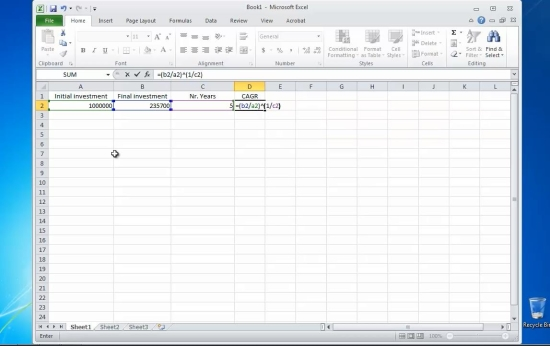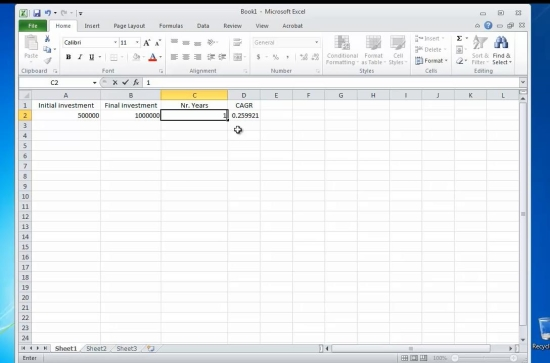## How to Calculate the Compound Annual Growth Rate, or CAGR, in Microsoft Excel

In this tutorial you will learn how to calculate the compound annual growth rate, or CAGR, in Microsoft Excel .Compound Annual Growth Rate is a financial statistical function that measures the rate of return on investment over a specified period of time.

Here is how to calculate it.

Prerequisites: Microsoft ExcelStep 2. Type in the value of initial amount of investment in the first cell – A

Type in the value of final amount of investment in the second cell – B

Type the number of years in the third cell – CStep 3. Go to the cell where you want to place the result, and type in the formula:

= (B/A)^(1/C)-1. Hit „ Enter”.Result: You have successfully calculated the Compound Annual Growth Rate in Excel.